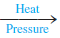# Problem: Urea (H2NCONH2) is used extensively as a nitrogen source in fertilizers. It is produced commercially from the reaction of ammonia and carbon dioxide:2NH3(g) + CO2(g)  H2NCONH2(s) + H2O(g)Ammonia gas at 223°C and 90. atm flows into a reactor at a rate of 500. L/min. Carbon dioxide at 223°C and 45 atm flows into the reactor at a rate of 600. L/min. What mass of urea is produced per minute by this reaction assuming 100% yield?

🤓 Based on our data, we think this question is relevant for Professor Velazquez Mojica's class at UCD.

###### FREE Expert Solution

We are asked to calculate the mass of urea is produced per minute by this reaction assuming 100% yield

2NH3(g) + CO2(g) →  H2NCONH2(s) + H2O(g)

Step 1. Calculate moles per minute of each

NH3

n = 1105.27 mol/min NH3

CO2

n = 663.16 mol/min CO2

Step 2. Simultaneously calculate the theoretical yield and identify the limiting reactant.

Use both to calculate for H2NCONH2

Molar mass H2NCONH= 60.07 g/mol###### Problem Details

Urea (H2NCONH2) is used extensively as a nitrogen source in fertilizers. It is produced commercially from the reaction of ammonia and carbon dioxide:

2NH3(g) + CO2(gH2NCONH2(s) + H2O(g)

Ammonia gas at 223°C and 90. atm flows into a reactor at a rate of 500. L/min. Carbon dioxide at 223°C and 45 atm flows into the reactor at a rate of 600. L/min. What mass of urea is produced per minute by this reaction assuming 100% yield?